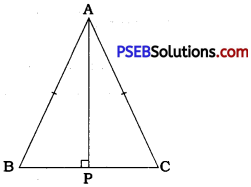# PSEB 9th Class Maths Solutions Chapter 7 Triangles Ex 7.3

Punjab State Board PSEB 9th Class Maths Book Solutions Chapter 7 Triangles Ex 7.3 Textbook Exercise Questions and Answers.

## PSEB Solutions for Class 9 Maths Chapter 7 Triangles Ex 7.3

Question 1.
∆ ABC and ∆ DBC are two isosceles triangles on the same base BC and vertices A and D are on the same side of BC (see the given figure). If AD is extended to intersect BC at D show that,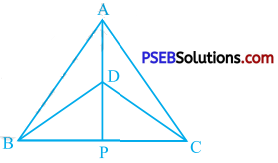(i) ∆ ABD ≅ ∆ ACD
(ii) ∆ ABP ≅ ∆ ACP
(iii) AP bisects ∠ A as well as ∠ D.
(iv) AP is the perpendicular bisector of BC.
∆ ABC and ∆ DBC are isosceles triangles on the same base BC.
∴ In ∆ ABC, AB = AC and in ∆ DBC, DB = DC.
In ∆ ABD and ∆ ACD,
AB = AC
DB = DC
∴ ∆ ABD s ∆ ACD (SSS rule) [Result (i)]
In ∆ ABP and ∆ ACP
AB = AC
and AP = AP (Common)
∴ ∆ ABP ≅ ∆ ACP (SAS rule) [Result (ii)]
∴ BP = CP (CPCT)
In ∆ DBP and ∆ DCR
DB = DC
BP = CP
and DP = DP (Common)
∴ ∆ DBP ≅ ∆ DCP (SSS rule)
From ∆ ABP ≅ ∆ ACR ∠ BAP = ∠ CAP (CPCT)
∴ AP bisects ∠A.
From ∆ DBP ≅ ∆ DCR ∠BDP = ∠ CDP (CPCT)
∴ DP bisects ∠D.
Thus, AP bisects ∠A as well as ∠D. [Result (iii)]
∆ A ABP ≅ ∆ ACP
∴ BP = CP and ∠ APB = ∠ APC (CPCT)
But, ∠ APB + ∠ APC = 180° (Linear pair)
∴ ∠ APB = ∠ APC =  = 90°
Thus, BP = CP and AP ⊥ BC.
∴ AP is the perpendicular bisector of BC. [Result (iv)]Question 2.
AD is an altitude of an isosceles triangle ABC in which AB = AC. Show that: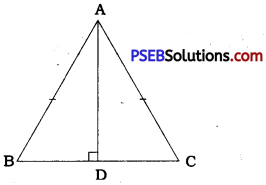AD is an altitude of A ABC.
hypotenuse AB = hypotenuse AC (Given)
Now, BD = CD means D is the midpoint of BC.
Hence, AD bisects BC. [Result (i)]
Hence, AD bisects ∠A. [Result (ii)]Question 3.
Two sides AB and BC and median AM of one triangle ABC are respectively equal to sides PQ and QR and median PN of ∆ PQR (see the given figure). Show that:
(i) ∆ ABM ≅ ∆ PQN
(ii) ∆ ABC ≅ ∆ PQR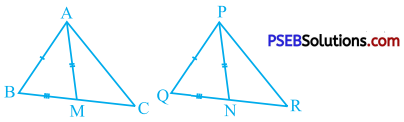In ∆ ABC, AM is a median.
∴ BM = CM = $$\frac{1}{2}$$ BC
In ∆ PQR, PN is a median.
∴ QN = RN = $$\frac{1}{2}$$ QR
Now, BC = QR (Given)
∴ $$\frac{1}{2}$$ BC = $$\frac{1}{2}$$ QR
∴ BM = QN
In ∆ ABM and ∆ PQN,
AB = PQ (Given)
AM = PN (Given)
BM = QN (Proved)
∴ ∆ ABM ≅ ∆ PQN (SSS rule) [Result (i)]
∴ ∠ ABM = ∠ PQN (CPCT)
∴ ∠ ABC = ∠ PQR
Now, in ∆ ABC and ∆ PQR,
AB = PQ
∠ ABC = ∠ PQR
BC = QR .
∴ ∆ ABC ≅ ∆ PQR (SAS rule) [Result (ii)]Question 4.
BE and CF are two equal altitudes of a triangle ABC. Using RHS congruence rule, prove that the triangle ABC is isosceles.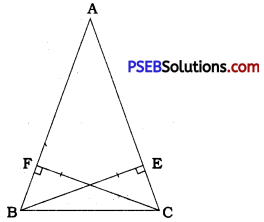In ∆ FBC and ∆ ECB,
CF = BE (Given)
∠ CFB = ∠ BEC = 90° (Given)
BC = CB (Common)
∴ A FBC ≅ A ECB (RHS rule)
∴ ∠ FBC = ∠ ECB (CPCT)
∴ ∠ ABC = ∠ ACB
Now, in ∆ ABC, ∠ ABC = ∠ ACB
∴ AC = AB (Theorem 7.3)
Hence, ∆ ABC is an isosceles triangle.Question 5.
ABC is an isosceles triangle with AB = AC. Draw AP ⊥ BC to show that ∠ B = ∠ C.